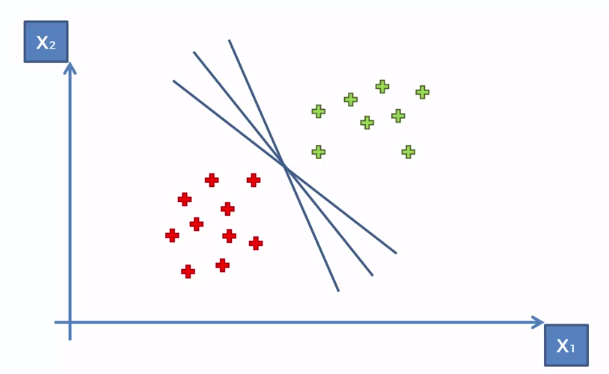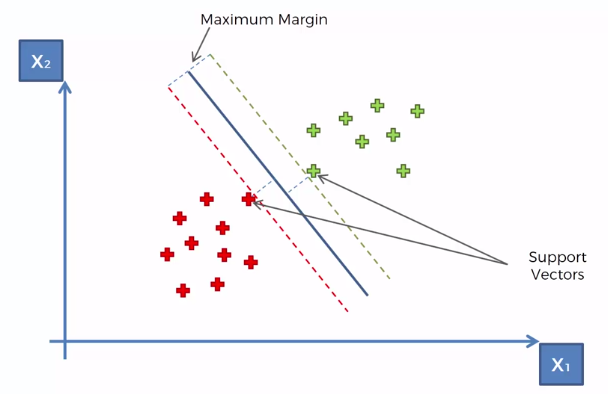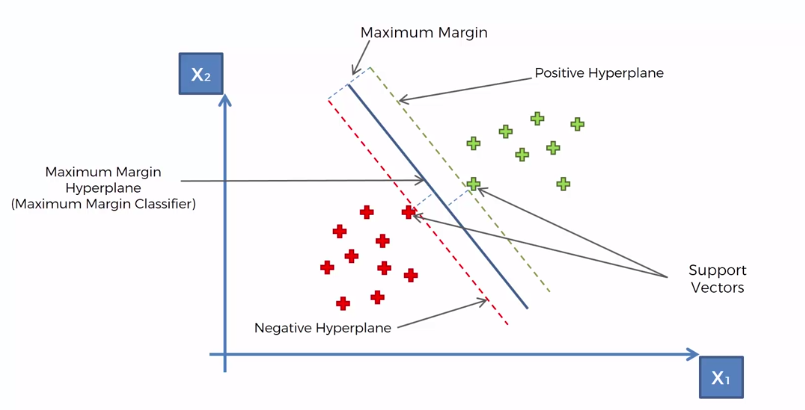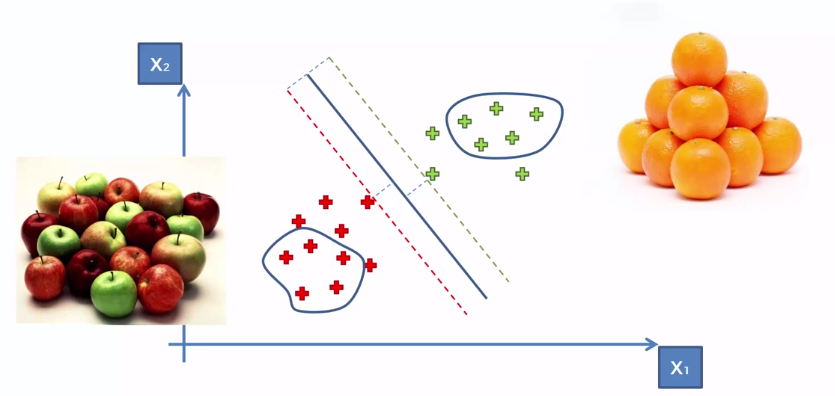## Introduction

The goal of support vector machines is to separate categorical data in the most optimal way, so that when a new data point is entered it can easily be classified.By creating a line of seperation we can easily predict where a new data point will be classified based upon where it lands in respect to the line. The line we end up choosing however is important because it determines what gets classified where.

Given training examples, each marked as belonging to one or the other of two categories, an SVM training algorithm builds an OPTIMAL model that assigns new examples to one category or the other, making it a non-probabilistic binary linear classifier.

## How the SVM works

The SVM works using the following logic. It takes the two points from each class that are closest to the opposing class. These points are called Support Vectors. The SVM can generate an optimal line by finding a point that is equidistant from the support vectors. This area between the support vectors is called the Maximum Margin and is used to classify new data.The support vector points are the only important points in the entire dataset for producing the Maximum Margin, meaning all points could disappear and it would not affect the SVM. The reason we call them vectors is because in a multidimensional space, we couldn’t visualize or make use of points, but vectors are a lot easier to work with mathematically.The line that’s produced itself is called Maximum Margin Hyperplane (hyperplane because of multidimensional space).

## Why and when to use SVMImagine trying to distinguish an apple from an orange. Typically,we give it a dataset of oranges and apples and normally a machine learning algorithm will look for features that make an apple an apple and an orange an orange.SVM uses the data from vectors closest to the Maximum Margin (support vectors), in other words SVM learns based off of apples that look the most like oranges and oranges that look most like apples.## Building an SVM

Use the classification template to set eveything up

Create the classifer

from sklearn.svm import SVC
classifier = SVC(kernel = 'linear', random_state = 0)
classifier.fit(X_train, y_train)


## Building an SVM (Not linearily seperable)

Create classifer

kernal : linear, ploy, rbf

from sklearn.svm import SVC
classifier = SVC(kernel = 'rbf', random_state = 0)
classifier.fit(X_train, y_train)